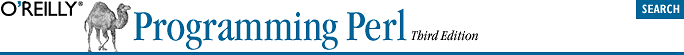home | O'Reilly's CD bookshelfs | FreeBSD | Linux | Cisco | Cisco ExamWhen an overloaded operator is, er, operated, the corresponding handler is invoked with three arguments. The first two arguments are the two operands. If the operator only uses one operand, the second argument is undef.

The third argument indicates whether the first two arguments were swapped. Even under the rules of normal arithmetic, some operations, like addition or multiplication, don't usually care about the order of their arguments, but others, like subtraction and division, do. Consider the difference between:

`\$object - 6`
and:
`6 - \$object`
If the first two arguments to a handler have been swapped, the third argument will be true. Otherwise, the third argument will be false, in which case there is a finer distinction as well: if the handler has been triggered by another handler involving assignment (as in += using + to figure out how to add), then the third argument is not merely false, but undef. This distinction enables some optimizations.

 Your overloaded objects are not required to respect the rules of normal arithmetic, of course, but it's usually best not to surprise people. Oddly, many languages make the mistake of overloading + with string concatenation, which is not commutative and only vaguely additive. For a different approach, see Perl.

As an example, here is a class that lets you manipulate a bounded range of numbers. It overloads both + and - so that the result of adding or subtracting objects constrains the values within the range 0 and 255:

```package ClipByte;

'-' => \&clip_sub;

sub new {
my \$class = shift;
my \$value = shift;
return bless \\$value => \$class;
}

my (\$x, \$y) = @_;
my (\$value) = ref(\$x) ? \$\$x : \$x;
\$value     += ref(\$y) ? \$\$y : \$y;
\$value = 255 if \$value > 255;
\$value =   0 if \$value < 0;
return bless \\$value => ref(\$x);
}

sub clip_sub {
my (\$x, \$y, \$swap) = @_;
my (\$value) = (ref \$x) ? \$\$x : \$x;
\$value     -= (ref \$y) ? \$\$y : \$y;
if (\$swap) { \$value = -\$value }
\$value = 255 if \$value > 255;
\$value =   0 if \$value < 0;
return bless \\$value => ref(\$x);
}

package main;

\$byte1 = ClipByte->new(200);
\$byte2 = ClipByte->new(100);

\$byte3 = \$byte1 + \$byte2;    # 255
\$byte4 = \$byte1 - \$byte2;    # 100
\$byte5 = 150 - \$byte2;       # 50```
You'll note that every function here is by necessity a constructor, so each one takes care to bless its new object back into the current class, whatever that is; we assume our class might be inherited. We also assume that if \$y is a reference, it's a reference to an object of our own type. Instead of testing ref(\$y), we could have called \$y->isa("ClipByte") if we wanted to be more thorough (and run slower).